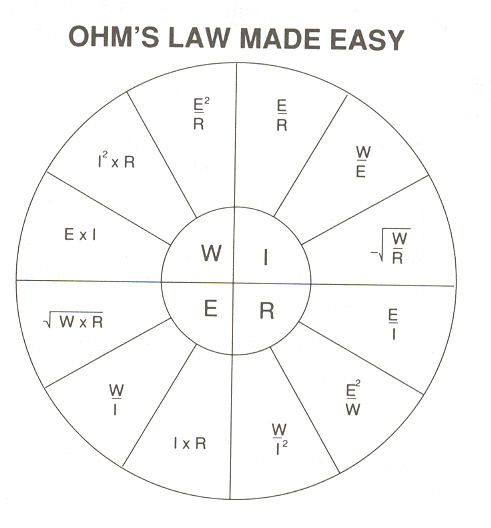# Electricity1 watt = 3.413 BTUs

1 kW = 1,000 watts = 3,413 BTUs

1 MW = 1,000 kW = 1,000,000 watts = 3,413,000 BTUs

95 to 100% efficient at THE POINT OF USE

746 watts = 1 Horse Power Mechanical

Fuel Cost Conversion Formulas:

1 CCF Natural Gas = about 100,000 BTUs / 3,413 BTUs/kWh = 29 kWh/CCF

Cost per kWh x 29 = Cost per CCF Excluding Efficiency Factors